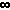Home    |    Teacher    |    Parents    |    Glossary    |    About UsEmail this page to a friendResources· Cool Tools · Formulas & Tables · References · Test Preparation · Study Tips · Wonders of MathSearchInterest and Exponential Growth (Math | General | Interest and Exponential Growth)

### The Compound Interest Equation

P = C (1 + r/n) nt
where
P = future value
C = initial deposit
r = interest rate (expressed as a fraction: eg. 0.06)
n = # of times per year interest is compounded
t = number of years invested

### Simplified Compound Interest Equation

When interest is only compounded once per year (n=1), the equation simplifies to:
P = C (1 + r) t

### Continuous Compound Interest

When interest is compounded continually (i.e. n -->), the compound interest equation takes the form:
P = C e rt

### Demonstration of Various Compounding

The following table shows the final principal (P), after t = 1 year, of an account initially with C = \$10000, at 6% interest rate, with the given compounding (n). As is shown, the method of compounding has little effect.
 n P 1 (yearly) \$ 10600.00 2 (semiannually) \$ 10609.00 4 (quarterly) \$ 10613.64 12 (monthly) \$ 10616.78 52 (weekly) \$ 10618.00 365 (daily) \$ 10618.31 continuous \$ 10618.37

### Loan Balance

Situation: A person initially borrows an amount A and in return agrees to make n repayments per year, each of an amount P. While the person is repaying the loan, interest is accumulating at an annual percentage rate of r, and this interest is compounded n times a year (along with each payment). Therefore, the person must continue paying these installments of amount P until the original amount and any accumulated interest is repaid. This equation gives the amount B that the person still needs to repay after t years.
 B = A (1 + r/n)NT - P (1 + r/n)NT - 1 (1 + r/n) - 1
where
B = balance after t years
A = amount borrowed
n = number of payments per year
P = amount paid per payment
r = annual percentage rate (APR)